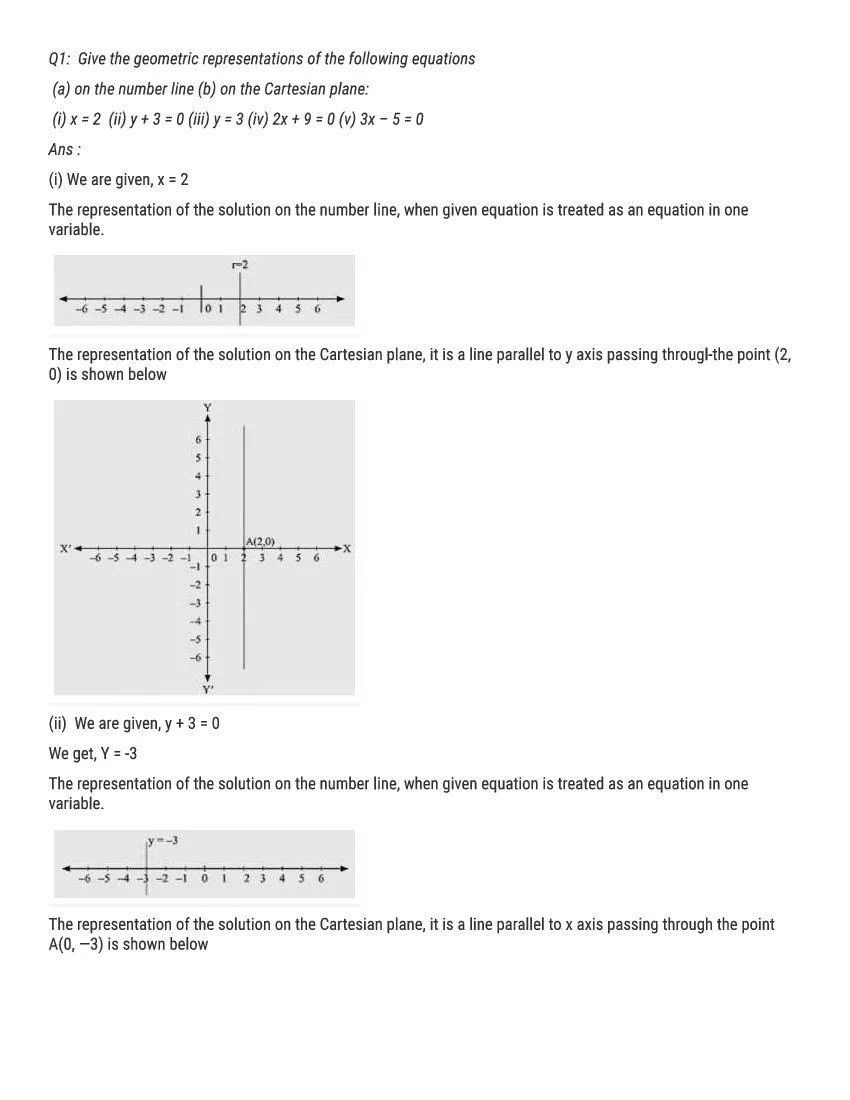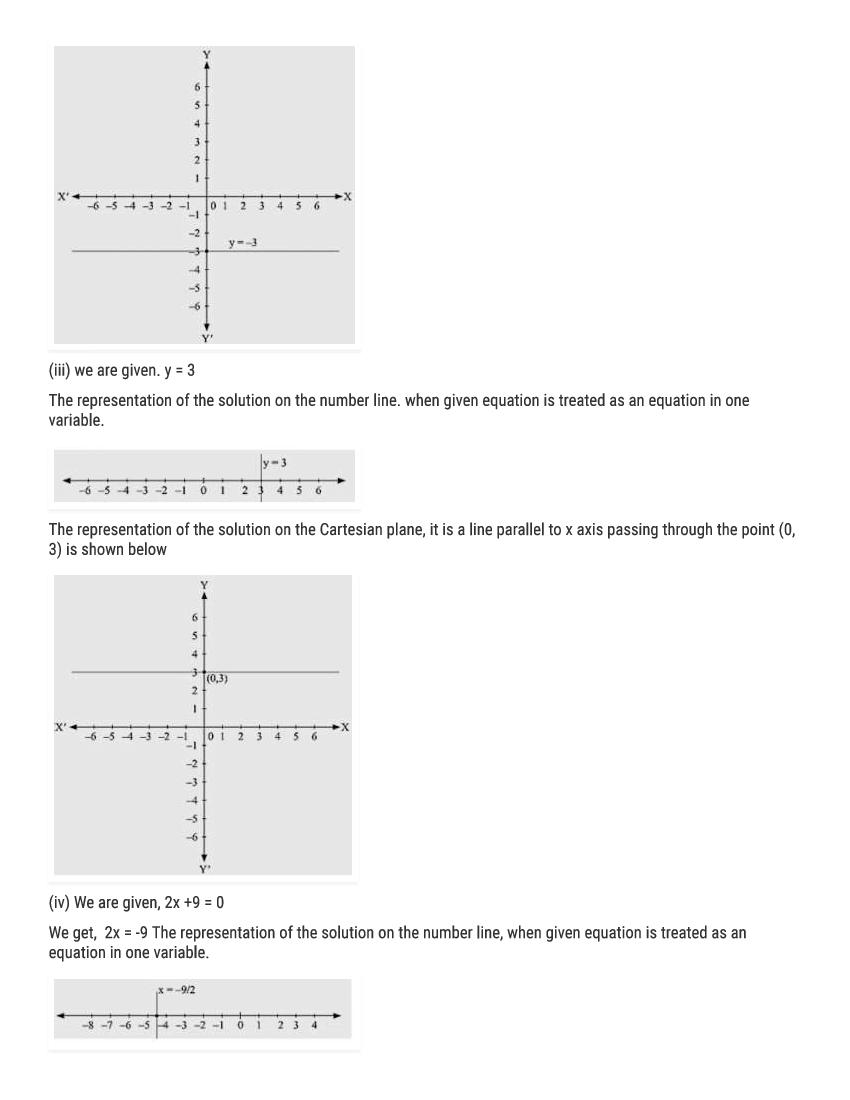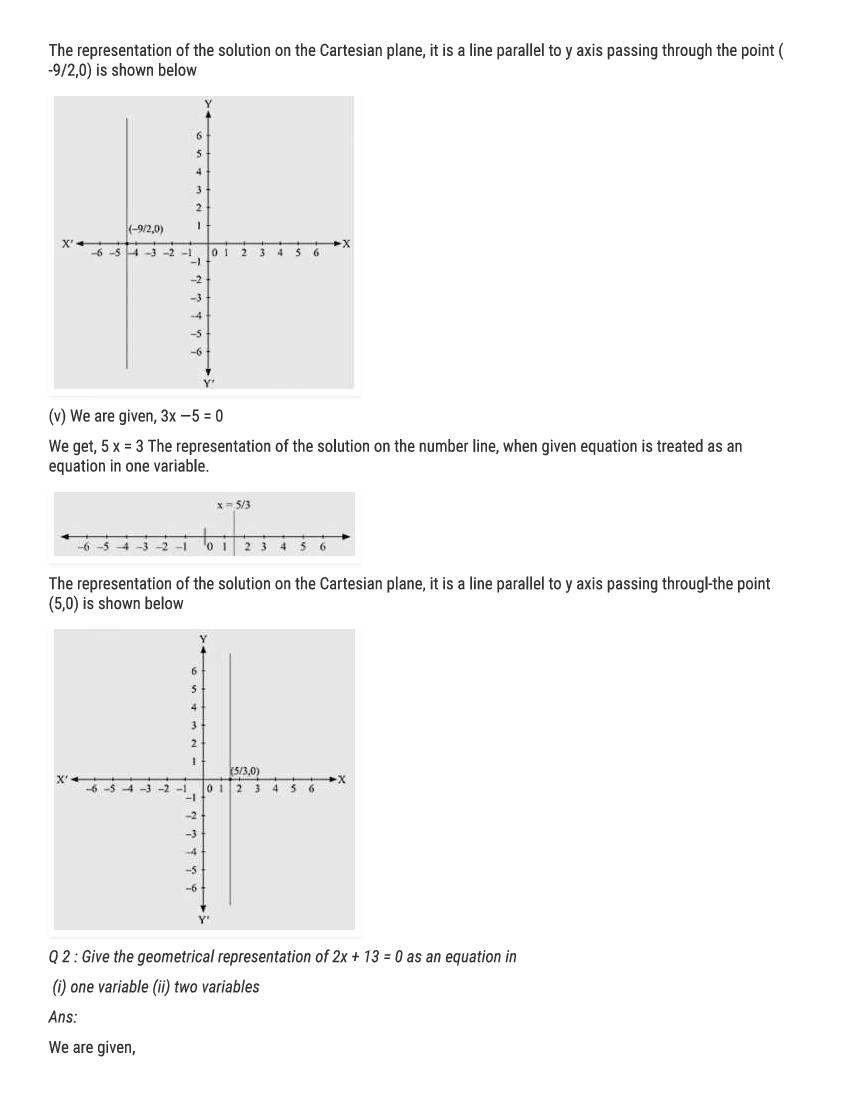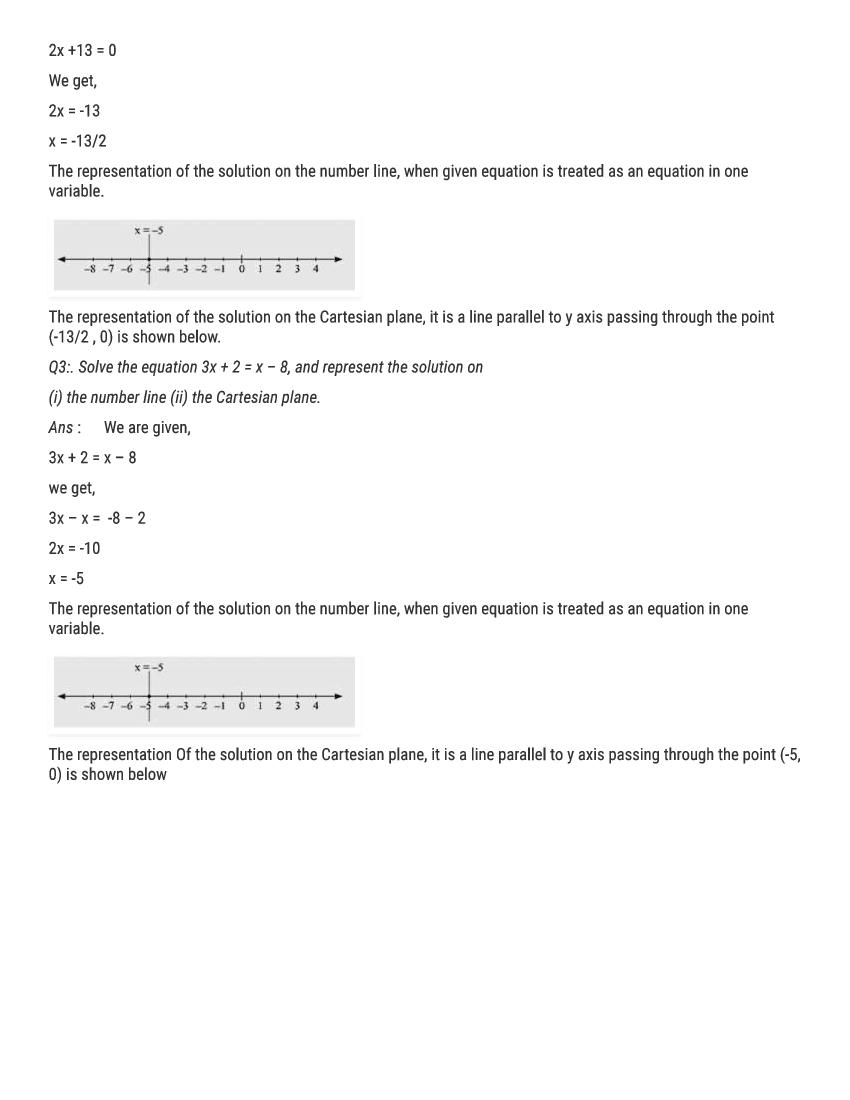# RD Sharma Solutions Class 9 Chapter 13 Linear Equation in Two Variables Exercise 13.4

Here you can get free RD Sharma Solutions for Class 9 Maths Chapter 13 Linear Equation in Two Variables Exercise 13.4. All RD Sharma Book Solutions are given here exercise wise for the chapter Linear Equation in Two Variables. RD Sharma Solutions are helpful in the preparation of several school level, graduate and undergraduate level competitive exams. Practicing questions from RD Sharma Mathematics Solutions for Class 9 Chapter 13 Linear Equation in Two Variables is proven to enhance your math skills.

 Class: Class 9th Chapter: Chapter 13 Exercise: Exercise 13.4 Name: Linear Equation in Two Variables

## RD Sharma Solutions Class 9 Chapter 13 Linear Equation in Two Variables Exercise 13.4

RD Sharma Class 9 Solutions Chapter 13 for Linear Equation in Two Variables Exercise 13.4 are given below.

RD Sharma Solutions Class 9 Chapter 13 Linear Equations in Two Variables Excercise 13.4RD Sharma Solutions for Class 9 Maths PDF download for Chapter 13 Linear Equation in Two Variables Exercise 13.4 is available here for free.

 « Previous Next »

### RD Sharma Solutions Class 9 Chapter 13 Exercise Wise

You can also see RD Sharma Solutions of all exercises of Chapter 13 – Linear Equation in Two Variables from here.

### RD Sharma Solutions Class 9 Chapter Wise

If you want RD Sharma solutions of any topic other than Chapter 13 Linear Equation in Two Variables, check it from here. Mathematics by RD Sharma has all chapters that are in your Class 9 syllabus.

Click to rate this post!
[Total: 1 Average: 5]